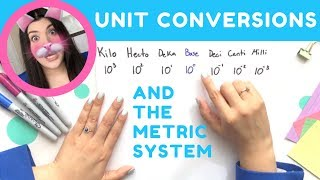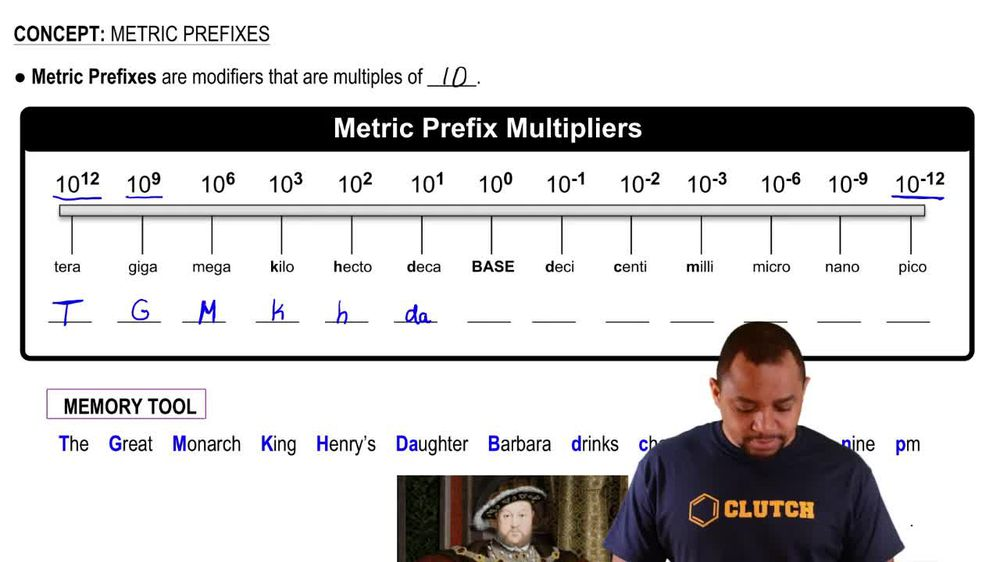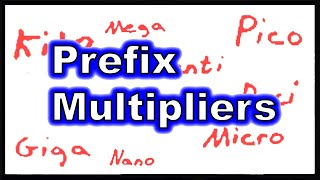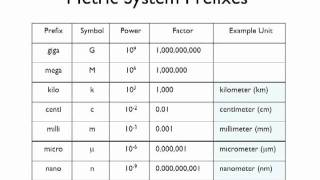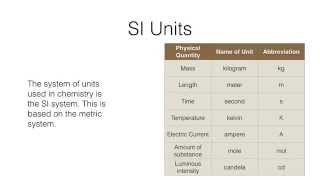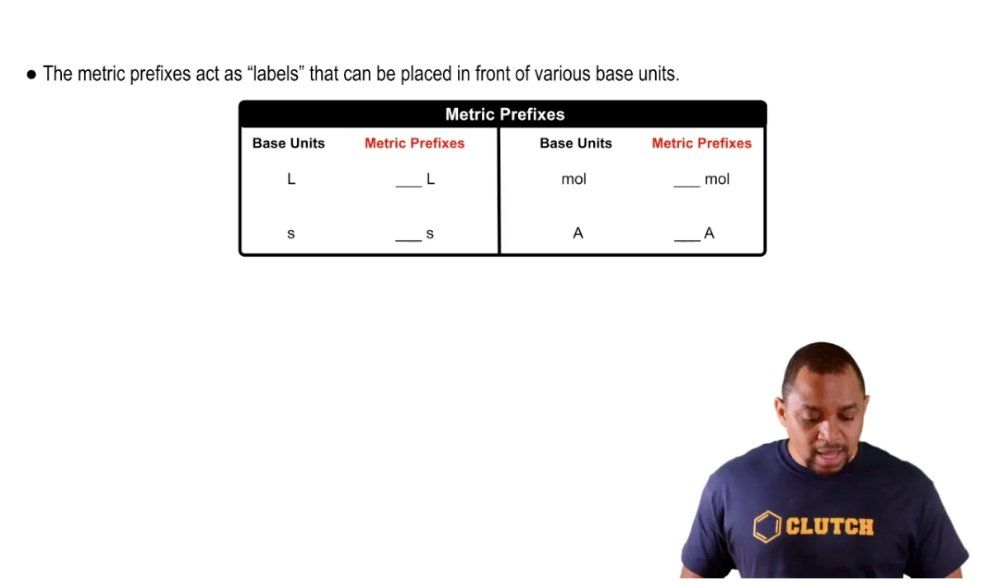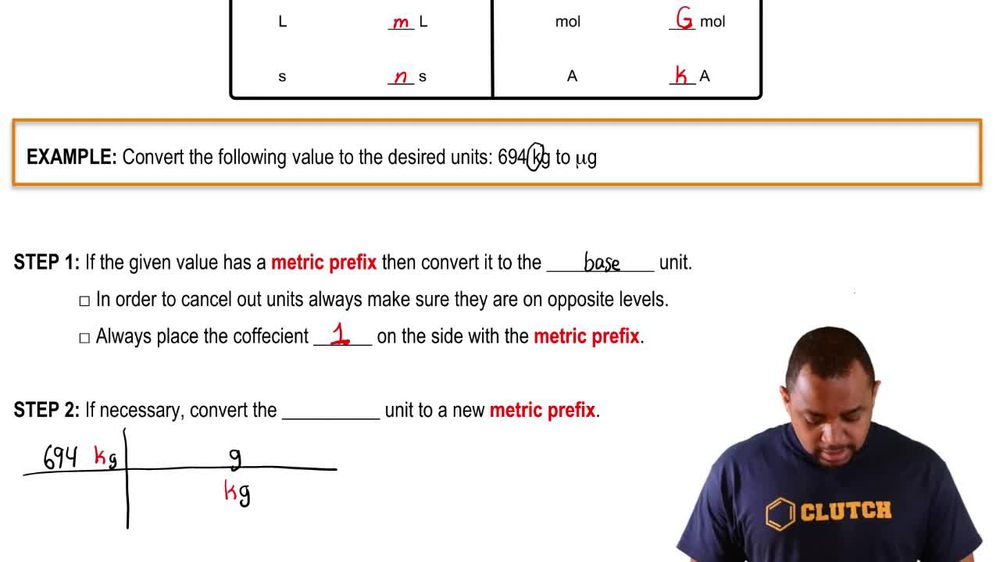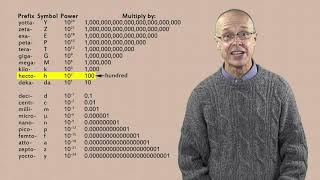Start typing, then use the up and down arrows to select an option from the list.
1. 1. Intro to General Chemistry2. Metric Prefixes
Problem

# How many microliters are in 1 L? In 20 mL?

Relevant Solution1m
Play a video:
Hello everyone today. We have the following problem determining nanoseconds in two seconds and 19 milliseconds. So for our first part we have two seconds. So it's important to note some common conversion factors. One is at one nanosecond is equal to 10 to the negative ninth seconds and one millisecond is equal to 10 to the negative third seconds so far. First part we have two seconds and so to get from seconds to nanoseconds we have to multiply by the conversion factor, that one nanosecond is equal to 10 to the negative ninth seconds. So that one argument to cancel the assembly left with nanoseconds. So we're gonna have two times 10 To the 9th nanoseconds for our first portion. For our second part we have 19 milliseconds and we want to go to nanoseconds. So first we're going to convert those milliseconds to regular seconds and say that one millisecond is equal to 10 to the negative 3rd seconds and then we're going to say that One nanosecond is equal to 10 to the negative 9th seconds. So once our units cancel out as they did before we left with 1.9 times 10 to the 7th milliseconds as our answer. And with that we've answered the question overall I hope this helped and until next time